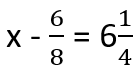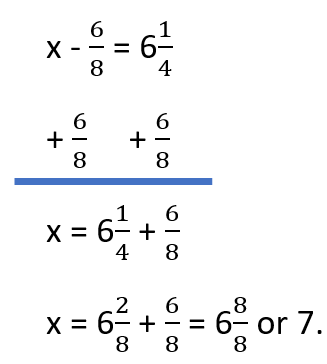# Math Worksheets Land

Math Worksheets For All Ages

# Math Worksheets Land

Math Worksheets For All Ages

Home > Math Topics > Algebra >

# Solving Equations with Fractions and Decimals Worksheets

Students seem to have no problem when working with equations that have standard numbers listed as their constants. We need to remember that constants are the values that never change, and they come in many different forms. They can be a fraction, decimal, letter, or even a symbol. In order to excel with algebra, you will need to first accept that these are the possibilities of what you will need to work with and learn how to manipulate equations to bend to your will regardless of the form that their constants take on. These worksheets are filled with problem that include basic equations that also have fractions and decimals as their constants.

### Aligned Standard: 6.EE.B.7

• Answer Keys - These are for all the unlocked materials above.

### Homework Sheets

This flows right in sync with the previous material.

• Homework 1 - Find the value of each variable. Just cancel out the values that bug you with the methods we have discussed.
• Homework 2 - Lots of fractions up in here. Reduce these values to see if that helps.
• Homework 3 - The variable change each step of the way. Make note of the changes that take place.
• Practice Worksheet 3 - A nice mix of decimals and fractions, but it is more decimal heavy.

### Math Skill Quizzes

Time to see if students really know their stuff.

• Quiz 1 - A lot of negative values that need a change of heart.
• Quiz 2 - Watch out for the blue number holder.
• Quiz 3 - This quiz is fraction heavy. Give it a few tries to make sure you have it down.

### Tips for Solving All Kinds of Algebraic Equations

Solving algebraic expressions is the first step towards learning advanced algebra. Thus, it requires the students to be in focus, given that one mistake could make render the entire solution wrong. To solve algebraic expressions perfectly, keep in mind the following tips stated below.

The basic strategy for solving an algebraic equation is first isolating the variable terms to one side of the equal sign. Then you start applying the inverse operations to those that are present to shake that variable out by itself. An inverse operation undoes another operation. The common examples are addition and subtraction, multiplication and division. If this operation is paired with a consonant, the constant must be included with the opposite operation. Always remember that any operation that is applied on one side of the equation should be applied on the other side as well, in order to maintain balance on both sides. If this rule is maintained, you can easily change the terms involved in the equation, without changing the relationship between them.

Firstly, if you have a variable mentioned only once in an equation, you can leave it on one side of the relational operator and take the numbers on the other side. For instance:

x + 3 = 5 | x = 5 - 3

Notice that the significant changes that occur when you decide to move the number to the other side. Now, the answer will be: x = 2.

### What About Constants That Are Fraction or Decimals?

Constants are values that do not change in equation, in most cases they are numeric values. They can also be a letter or a symbol too like the constants e (Euler’s number) and π (Pi). Just like we showed you in the last example, constants do not change, so you would just treat decimals and fractions as any other numbers and perform the counter operations. Take a look at an example of each.

Decimal Example Problem: 0.6x = 13.8

Solution: To solve this problem, we will need to get the variable (x) by itself. Identify the operations and values being applied to the variable (x). Apply the inverse operations and values to both sides of the equals sign. 0.6 and x are being multiplied. The opposite of multiplication is division. Since x is being multiplied by 0.6. We will divide both sides of the equal sign by 0.6.

The math: (0.6x) ÷ 0.6 = x, 13.8 ÷ 0.6 = 23. This leaves us with x =23.

Decimal Example Problem: 0.6x = 13.8

Solution: To solve this problem, we will need to get the variable (x) by itself. Identify the operations and values being applied to the variable (x). Apply the inverse operations and values to both sides of the equals sign. 0.6 and x are being multiplied. The opposite of multiplication is division. Since x is being multiplied by 0.6. We will divide both sides of the equal sign by 0.6.

The math: (0.6x) ÷ 0.6 = x, 13.8 ÷ 0.6 = 23. This leaves us with x =23.

Fraction Example Problem:Solution: Our goal with this problem is to get the variable (x) by itself. The easiest way to do this is to see what operations are being performed on the side of equal sign that x is on and just perform the exact opposite operation to both sides of the equal symbol. In this case 6/8 is being subtracted from the variable (x) and that is the only operation taking place, so if we add 6/8 to both sides, we will get x by itself. We can rewrite 1/4 as 2/8 and then complete the problem.

The math:### How to Approach Problems That Include Fractions and Decimals

When you first begin to see problems that include a fraction or decimal your first goal should be to try to neutralize these values to make your operations simpler. When it comes to fraction focus on finding common factors of both the numerator and denominator are reduce that value into the simplest form. Once that value is reduced rearrange the equation to get the variable by itself. If the fraction is still difficult to work with remember that you can use the reciprocal to make it basically disappear, as needed. Just remind yourself that you need to process this operation on both sides of the equals symbol. When working with decimals it helps to just get rid of the decimal form by multiplying it by a whole number value. For example, if you had a value that ends in two-tenths, multiplying everything by five would make that decimal a whole number value. Once again, make sure that you apply this product to every term in the equation and you will be good to go. As with everything repetition is the key to getting good at this skill.

Unlock all the answers, worksheets, homework, tests and more!
Save Tons of Time! Make My Life Easier Now

## Thanks and Don't Forget To Tell Your Friends!

I would appreciate everyone letting me know if you find any errors. I'm getting a little older these days and my eyes are going. Please contact me, to let me know. I'll fix it ASAP.